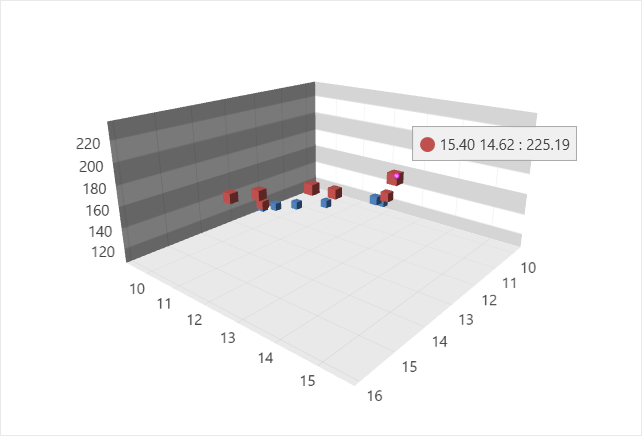Returns a collection of calculated fields.

Namespace: DevExpress.Xpf.Charts

Assembly: DevExpress.Xpf.Charts.v23.1.dll

NuGet Package: DevExpress.Wpf.Charts

## Declaration

``public ChartCalculatedFieldCollection CalculatedFields { get; }``

## Property Value

Type Description
ChartCalculatedFieldCollection

A collection that stores calculated fields.

## Remarks

Calculated fields allow you to evaluate values based on an expression and use them as a data source for series arguments, values, crosshair labels, and so on.

To add a calculated field to a Chart3D Control, add a ChartCalculatedField object to the adapter’s CalculatedFields collection and specify its parameters.

## Example

The following example shows how to create a calculated field and then use this field as a data source for a series. The field’s values are calculated by the following expression: [X] * [Y].``````<dxc:Chart3DControl AspectRatio="1 1 0.5">
<dxc:Chart3DControl.DataContext>
<local:ChartViewModel/>
</dxc:Chart3DControl.DataContext>
XArgumentDataMember="X"
YArgumentDataMember="Y"
ValueDataMember="Value"
DataSource="{Binding DataPoints}">
<dxc:ChartCalculatedField FieldName="Value"
Expression="[X]*[Y]"
FieldType="Double"/>